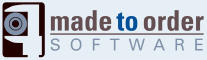# Sample Usage

The jsMath module is now a filter. By default, the filter detects all the delimiters as defined by the jsMath library. This includes $$...$$, $...$, $$...$$, $...$. It also supports the tag like syntax with $,$ and [display], [/display].

The $tag, parenthesis and single dollar sign generate an inline math equation. This means the result is saved in a <span> tag. The [display] tag, square brackets and double dollar sign generate a block with the math equation. By default, that block is centered which is the default way of presenting equations. This means the result is saved in a <div> tag. The double dollar sign delimiter can be changed to generate inline math equations. What is written between the tags is the usual TeX math equation. For instance, [math]\sqrt{x^2+1}$ and $\root 3\of {1-\pi x^2}$ generate the following equations:Very practical if you are writing a lot of mathematical equations!